Deutsche VersionCalculations:  voltage divider or potentiometer
Voltage drop at the voltage divider

 Using impedance matching or power matching you make the output impedance of a source equal to the input impedance of the load to which it is ultimately connected. T- and H-pads are used in radio frequency (RF) circuits to attenuate a signal (damping). It is applied where maximum energy (power) is transferred between a source and a load. Then Zsource = Zload.   But in sound recording (audio), public address, and HiFi only impedance bridging is used with:   Zsource << Zload or ZS << ZL or Zout << Zin   The output impedance of the source is always much smaller than the input impedance of the load. In this case never try to calculate and use T- and H-pads circuits - better use voltage dividers instead.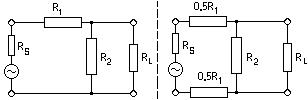asymmetric                   symmetric   Historical reasons show impedance values especially of 50 ohms, 200 ohms, or 600 ohms. Voltage Divider Calculator No. 1   Entering three or four values calculate the others. The value of Zload can be entered additionally, otherwise it uses automatically a 1 megohm load - unloaded open circuit. Use the left mouse button - click at a free space.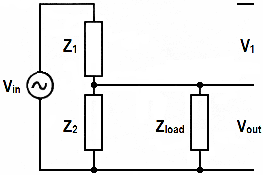Vin volts Z1 ohms Z2 ohms Vunloaded volts ZL ohms output voltage Vout volts

Vunloaded means Vout without ZL. If wanted, Zsource of the generator can be added to Z1.

 Voltage damping D = 20 × log10 ( Vout ) dB ------ --------------- Vin

A negative solution means damping (loss) - positive solution means amplification (gain).

Voltage damping: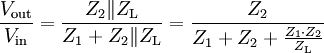Output voltage: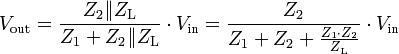Parallel resistance: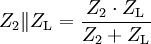Rule of thumb: The voltages are proportional to the resistances.

Formulas for the unloaded voltage divider: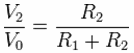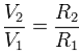Voltage Divider Calculator No. 2

 This calculator, given any three or four of the five possible values, will give the results for the remaining one. The ZL value is optional, if not supplied, the calculator uses 1 Megohm. Usefull if you need to enter Vout. Fill in any three or four fields in the form below, then click the "calculate" button. The remaining field will be calculated, and the results displayed. If you make a new calculation, use always the "reset" button to clear all the boxes. Z = R.Vin volts Vout volts Z1 ohms Z2 ohms ZL ohms
 To compute Input Voltage enter Z1, Output Voltage, and Z2 and then click the calculate button. To compute Z1 enter Input Voltage, Output Voltage, and Z2 and then click the calculate button. To compute Z2 enter Input Voltage, Output Voltage, and Z1 and then click the calculate button. To compute Output Voltage enter Input Voltage, Z1 and Z2 and then click the calculate button. If wanted, Zsource of the generator can be added to Z1.

Voltage division ratioα = Ratio (Output Voltage to Input Voltage) = Vout / Vin
Z2 = (α × Z1) / ( 1 − α)
dB (level) = 20 × log α
Vout = Vin × [Z2 / (Z1 + Z2)]

Voltage divider (potentiometer) with different control characteristics
Figure: © Detlef Mietke − http://www.elektroniktutor.de/analog/u_teiler.html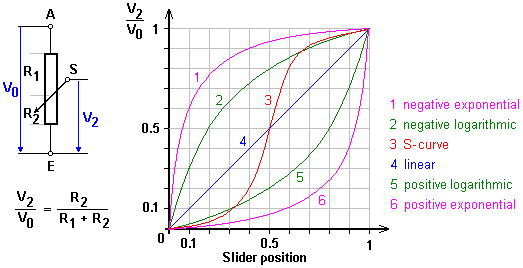Interconnection of two audio units equals a circuit of a voltage divider − Z2 << Z1.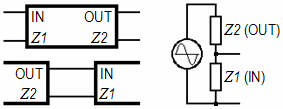Attention: The numbering of the impedances is inversely to a voltage divider.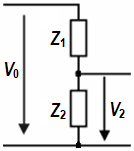A panpot is made of two voltage dividers:In sound engineering there is no Impedance matching or Power matching. In audio we use only (high) Impedance bridging or Voltage bridging.